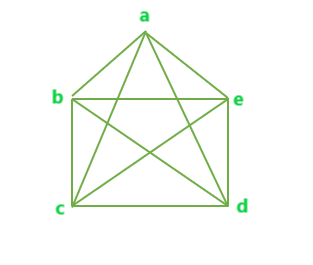# Program to find total number of edges in a Complete Graph

• Difficulty Level : Basic
• Last Updated : 08 Jun, 2022

Given N number of vertices of a Graph. The task is to find the total number of edges possible in a complete graph of N vertices.
Complete Graph: A Complete Graph is a graph in which every pair of vertices is connected by an edge.
Examples

```Input : N = 3
Output : Edges = 3

Input : N = 5
Output : Edges = 10```

The total number of possible edges in a complete graph of N vertices can be given as,

Total number of edges in a complete graph of N vertices = ( n * ( n – 1 ) ) / 2

Example 1: Below is a complete graph with N = 5 vertices.The total number of edges in the above complete graph = 10 = (5)*(5-1)/2.
Below is the implementation of the above idea:

## C++

 `// C++ implementation to find the``// number of edges in a complete graph` `#include ``using` `namespace` `std;` `// Function to find the total number of``// edges in a complete graph with N vertices``int` `totEdge(``int` `n)``{``    ``int` `result = 0;` `    ``result = (n * (n - 1)) / 2;` `    ``return` `result;``}` `// Driver Code``int` `main()``{``    ``int` `n = 6;` `    ``cout << totEdge(n);` `    ``return` `0;``}`

## Java

 `// Java implementation to find the``// number of edges in a complete graph` `class` `GFG {``    ` `// Function to find the total number of``// edges in a complete graph with N vertices``static` `int` `totEdge(``int` `n)``{``    ``int` `result = ``0``;` `    ``result = (n * (n - ``1``)) / ``2``;` `    ``return` `result;``}` `    ``// Driver Code``    ``public` `static` `void` `main(String []args)``    ``{``        ``int` `n = ``6``;``        ``System.out.println(totEdge(n));``    ``}` `}`

## Python 3

 `# Python 3 implementation to ``# find the number of edges``# in a complete graph` `# Function to find the total``# number of edges in a complete``# graph with N vertices``def` `totEdge(n) :` `    ``result ``=` `(n ``*` `(n ``-` `1``)) ``/``/` `2` `    ``return` `result``            ` `# Driver Code``if` `__name__ ``=``=` `"__main__"` `:` `    ``n ``=` `6` `    ``print``(totEdge(n))` `# This code is contributed``# by ANKITRAI1`

## C#

 `// C# implementation to find``// the number of edges in a``// complete graph``using` `System;` `class` `GFG``{``    ` `// Function to find the total``// number of edges in a complete``// graph with N vertices``static` `int` `totEdge(``int` `n)``{``    ``int` `result = 0;` `    ``result = (n * (n - 1)) / 2;` `    ``return` `result;``}` `// Driver Code``public` `static` `void` `Main()``{``    ``int` `n = 6;``    ``Console.Write(totEdge(n));``}``}` `// This code is contributed``// by ChitraNayal`

## PHP

 ``

## Javascript

 ``

Output:

`15`

Time Complexity: O(1)
Auxiliary Space: O(1)

My Personal Notes arrow_drop_up